# Time difference in excel & Google Sheets (Hours, Mins, Secs)

This tutorial will demonstrate how to calculate time differences in Excel and Google Sheets.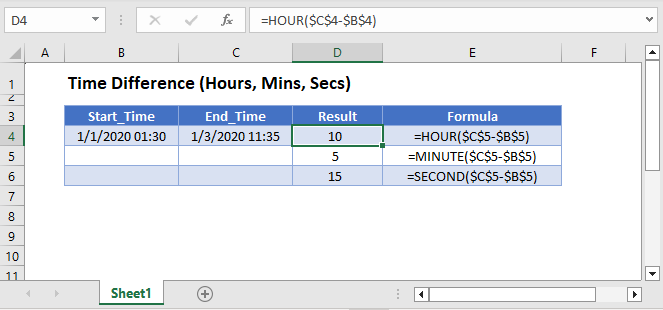## Calculate Total Time Difference

First, we will demonstrate how to calculate the total time difference between times in Hours, Minutes, or Seconds.

### Hours Between Times

If you need to calculate the total difference between two times, you can simply subtract the two times and multiply the result by 24:

``=(C3-B3)*24``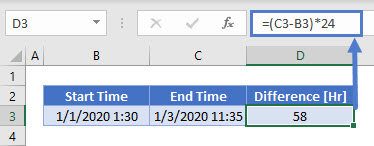### Minutes Between Times

If you need to calculate the total difference in minutes, you can subtract the times and multiply the result by 1440 (24*60).

``=(C3-B3)*1440``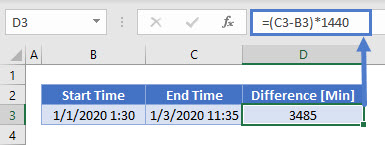### Seconds Between Times

Last, if you need to calculate the total difference between two times in seconds, multiply the result by 86400 (24*60*60).

``=(C3-B3)*86400``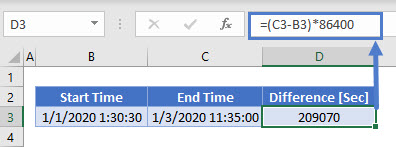## HOUR, MINUTE, and SECOND Functions

If you want to calculate the time difference, ignoring dates, there are several functions you can use:

## HOUR Function

With the HOUR Function you can calculate the difference in hours between two times.

``=HOUR(C3-B3)``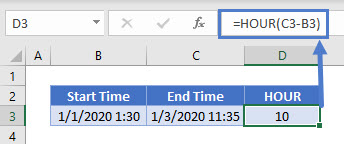### Easy Excel Automation## MINUTE Function

You can use the MINUTE function to calculate the difference in minutes between two times.

``=MINUTE(C3-B3)``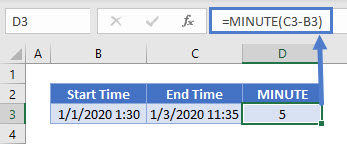## SECOND Function

With the SECOND function you can get the difference in seconds between two times:

``=SECOND(C3-B3)``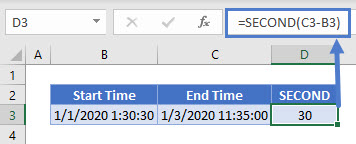## Time Difference in [h]:mm:ss Format

If you need to display the total time difference in hours, minutes and seconds, you can simply subtract the times and change the cell number formatting (Format Cells > Custom).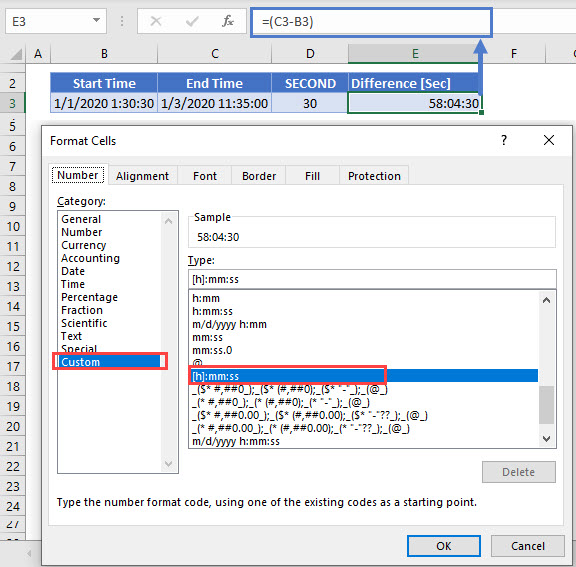## Time Difference in Google Sheets

These formulas work exactly the same in Google Sheets as in Excel.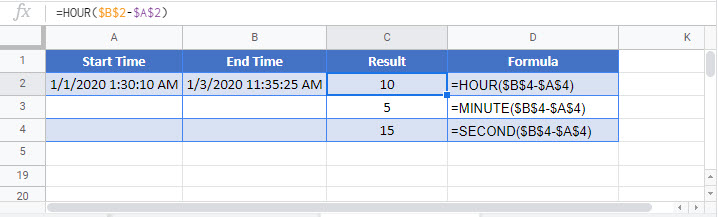### Excel Practice Worksheet

Practice Excel functions and formulas with our 100% free practice worksheets!